# Biasing MOSFET follower

#### PeteHL

Joined Dec 17, 2014
431
(In the circuit in the below schematic) there is a big discrepancy in voltage drop across the source resistor R3 according to simulation with Electronic Workbench (student edition) and what I measure at my actual, physical workbench. As this is such a simple circuit, I doubt that I have connected this up incorrectly at my bench.

So can anyone explain why simulated and actual voltages are so different?

I could comment some more at what I have been trying to do, but for now I'll leave it at that.

Thank you,
Pete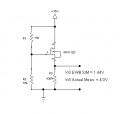#### Sitara

Joined May 2, 2014
57
Hi,
I don't have Electronics Workbench, but I do have LTSpice. Unfortunately, IRFD120 is not included in the LTSpice standard library for mosfets, nor could I find a spice model for it upon Googling for one. I did find a spice model for the IRF520, whose specs are a close match for the IRFD120 (see here: http://www.vishay.com/mosfets/v-ds-gteq-81-v-lteq-250-v/ ). On running the LTSpice Sim with your circuit values, I get a voltage of 3.75v across the source resistor, see screen dump below: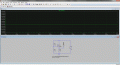Based on this result I suspect that the Electronics Workbench model for the IRFD120 is faulty.

Last edited:

#### #12

Joined Nov 30, 2010
18,224
True. Id/Vgs (current/control voltage) is so much more variable in mosfets than bi-polar transistors that most designs try to avoid dealing with it by using the mosfet as a hard switch and over driving the gate. Analog designs try to compensate for the uncertainty of the necessary gate voltage by allowing for a lot of range for the control voltage and controlling the output current or voltage with a feedback design.

•DickCappels

#### PeteHL

Joined Dec 17, 2014
431
Sitara and #12, thanks for your responses.

What I have been trying to do is bias so that

quiescent Vgs > threshold Vgs

for the purpose of insuring linear AC amplification. If quiescent Vgs is less than or equal to threshold Vgs, then amplification is not linear.

However, in testing different biasing voltage division of R2/(R1+R2) and resistance of source resistor R3, it seems to be not possible to achieve Vgs > 4V, where 4V is the maximum threshold voltage for IRFD120.

In fact, in my experimenting, Vgs is very nearly constant at about 3.5V no matter what the gate voltage (w.r.t. ground) and resistance of the source resistor are set at.

Last edited:

#### crutschow

Joined Mar 14, 2008
31,581
in my experimenting, Vgs is very nearly constant at about 3.5V no matter what the gate voltage (w.r.t. ground)
It is normal for the Vgs to remain close the Vth.
It only varies by the drain current divided by the MOSFET transconductance.

#### #12

Joined Nov 30, 2010
18,224
I'm not educated enough to relate to what crutschow just said, but another way to look at it is that you have designed the equivalent of an emitter follower. The higher you raise the gate voltage, the higher the voltage becomes at the top of R3. The circuit is fighting every increase in gate voltage by increasing the drain current and therefore source voltage. If you have a mosfet with a strong current capability (compared to R3), a small change in Vgs will cause a big change in Id. The result is that the voltage on R3 will chase the gate voltage all the way up to the power supply, if you keep raising the gate voltage.

After I wrote that, I can start to understand what crutschow said. He said it math terms. I said it more like a technician...because I am a technician.

#### PeteHL

Joined Dec 17, 2014
431
It is normal for the Vgs to remain close the Vth.
It only varies by the drain current divided by the MOSFET transconductance.
Thank you. Drain current in the circuit is << 1 amp and Vgs(th) equals 0.8, so that would be only a very small change of Vgs. Either I have misread about how to bias the voltage follower or the author is mistaken.

#### PeteHL

Joined Dec 17, 2014
431
I'm not educated enough to relate to what crutschow just said, but another way to look at it is that you have designed the equivalent of an emitter follower. The higher you raise the gate voltage, the higher the voltage becomes at the top of R3. The circuit is fighting every increase in gate voltage by increasing the drain current and therefore source voltage. If you have a mosfet with a strong current capability (compared to R3), a small change in Vgs will cause a big change in Id. The result is that the voltage on R3 will chase the gate voltage all the way up to the power supply, if you keep raising the gate voltage.

After I wrote that, I can start to understand what crutschow said. He said it math terms. I said it more like a technician...because I am a technician.
There is negative feedback that if I fully understood it, would probably help in designing the follower.

#### #12

Joined Nov 30, 2010
18,224
There is negative feedback
Definitely. Positive volts on the source = negative feedback compared to positive volts on the gate.
You can use the same principle for bipolar transistors (and vacuum tubes) by designing an in-phase feedback to arrive at the emitter (or cathode).

#### crutschow

Joined Mar 14, 2008
31,581
Below is an LTspice simulation to illustrate what I stated in post #5.
Note how the source voltage stays zero until the MOSFET Vgs threshold voltage of about 2.5V is reached (for the MOSFET I used).
Then the source voltage rises essentially with the same slope as Vg.
From that point on the Vgs voltage varies very little from the start of the output slope to its end (10.6mV measured), which is due to the transconductance gain of the MOSFET (about 8.9S for this MOSFET at that current level).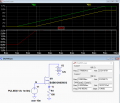Last edited:

#### Bordodynov

Joined May 20, 2015
3,040

#### ian field

Joined Oct 27, 2012
6,536
(In the circuit in the below schematic) there is a big discrepancy in voltage drop across the source resistor R3 according to simulation with Electronic Workbench (student edition) and what I measure at my actual, physical workbench. As this is such a simple circuit, I doubt that I have connected this up incorrectly at my bench.

So can anyone explain why simulated and actual voltages are so different?

I could comment some more at what I have been trying to do, but for now I'll leave it at that.

Thank you,
Pete
View attachment 124148
Did you take into account the VGSthr?

The possible parameter spread isn't small.

#### PeteHL

Joined Dec 17, 2014
431
Did you take into account the VGSthr?

The possible parameter spread isn't small.
The simulated transistor I would think would be given an average value of Vgs(th). In the worst case, the simulated transistor would have Vgs(th) equal to 2, and Vgs(th) of the transistor in my built circuit equal to 4, or vice versa. Could the worst case result in that big of a difference of voltage drop across the source resistor?

#### PeteHL

Joined Dec 17, 2014
431
Here is a biasing scheme that I cooked up, and the results shown in the attached circuit diagram.

(1) From the spec. sheets for IRFD120, Vgs(th)max. = 4V. So the gate voltage w.r.t. ground optimally would be

Vg = 0.5*[Vdd - Vgs(th)max] + Vgs(th)max. = 9.5V

This will result where R1 = 10k Ohm and R2 = 18k Ohm.

(2) Looking at the typical output characteristics plot in the spec. sheets, note that where Vgs is less than or equal to 4.5V, then Vds greater than or equal to 2V puts operation of the FET in the saturation region. Knowing this then,where Vdsq is quiescent Vds,

Vdsq = 0.5*[Vdd - 2V] + 2V = 8.5V

(3) In this second to last step I get uncouth, so all of you engineers had better look away now. Where there is no input signal,

Vr3 = Vdd - Vdsq = 15V - 8.5 = 6.5V

So by trial and error, make resistance of R3 such that there is a 6.5V drop across it. That turns out to happen when R3 = 1k Ohm.

(4) Power dissipation of the FET is given as 1.3W. So check that the follower doesn't result in greater dissipation than this.

P = Vdsq *Vr3/ R3 = 8.5V * 6.5mA = 60 mW (way below what the FET can handle)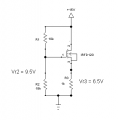#### PeteHL

Joined Dec 17, 2014
431
Did you take into account the VGSthr?

The possible parameter spread isn't small.
According to the spec sheets for IRFD120, the range of Vgs(th) is from 2V to 4V. In the worst case scenario of the simulated FET with Vgs(th) = 2V, and the FET in my built circuit with Vgs(th)= 4V, or vice versa, could that produce the ratio of voltage drop across the source resistor that I got, almost 3:1?

#### PeteHL

Joined Dec 17, 2014
431
Thanks for your simulation of Post #10, crutschow. If I am interpreting it correctly, I believe that the graphs shows that so long as Vg > Vgs(th) then if the changing gate voltage is considered to be an input signal, then the changing output signal exactly follows input.

#### #12

Joined Nov 30, 2010
18,224
then the changing output signal exactly follows input.
Not quite exactly, but very close.

#### crutschow

Joined Mar 14, 2008
31,581
if the changing gate voltage is considered to be an input signal, then the changing output signal exactly follows input.
Yes, in the simulation there was only about 10mV difference in the slope between the input and the output.
That's why it's called a source follower.Last edited:
•#12

#### DickCappels

Joined Aug 21, 2008
9,632
•#12

#### crutschow

Joined Mar 14, 2008
31,581
Dick, that's an interesting, low-offset source follower circuit.
I had to simulate it for grins.It shows an offset of less than a mV (for the ideal matched simulated BJTs).

In a real circuit it should stay within a few tens of millivolts or less.
Using an inexpensive matched NPN pair, such as this, would minimize the offset.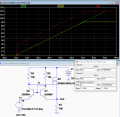#### Attachments

• 1.3 KB Views: 8
Last edited:
•DickCappels